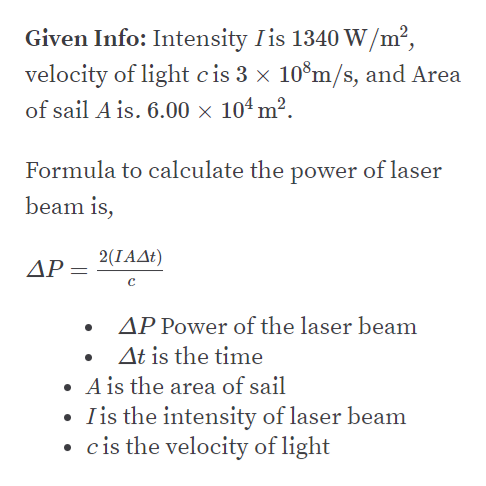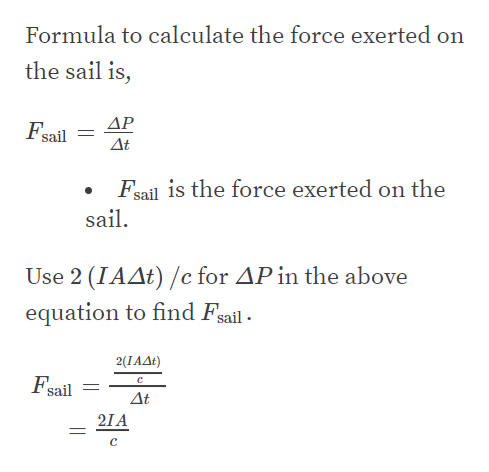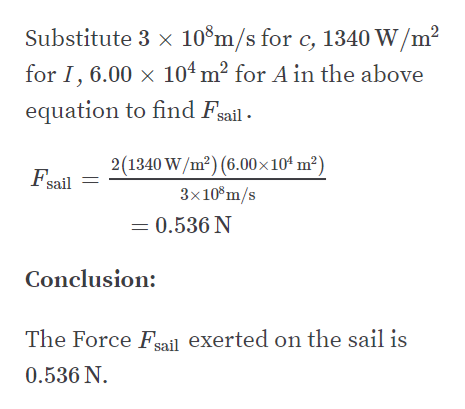# One possible means of achieving space flight is to place aperfectly reflecting aluminized sheet into Earth’s orbit andto use the light from the Sun to push this solar sail. Supposesuch a sail, of area 6.00 x 104 m2 and mass 6.00 x 103 kg,is placed in orbit facing the Sun. (a) What force is exertedon the sail? (b) What is the sail’s acceleration? (c) How longdoes it take this sail to reach the Moon, 3.84 x 108 m away?Ignore all gravitational effects and assume a solar intensity of1 340 W/m2. Hint: The radiation pressure by a reflected waveis given by 2 (average power per unit area)/c.

Question
8 views

One possible means of achieving space flight is to place a
perfectly reflecting aluminized sheet into Earth’s orbit and
to use the light from the Sun to push this solar sail. Suppose
such a sail, of area 6.00 x 104 m2 and mass 6.00 x 103 kg,
is placed in orbit facing the Sun. (a) What force is exerted
on the sail? (b) What is the sail’s acceleration? (c) How long
does it take this sail to reach the Moon, 3.84 x 108 m away?
Ignore all gravitational effects and assume a solar intensity of
1 340 W/m2. Hint: The radiation pressure by a reflected wave
is given by 2 (average power per unit area)/c.

check_circle

Step 1

Part A:help_outlineImage TranscriptioncloseGiven Info: Intensity Iis 1340 W/m², velocity of light cis 3 × 10°m/s, and Area of sail A is. 6.00 x 104 m². Formula to calculate the power of laser beam is, 2(ΙΑΔ ) ΔΡ= AP Power of the laser beam At is the time • A is the area of sail Iis the intensity of laser beam • cis the velocity of light fullscreen
Step 2help_outlineImage TranscriptioncloseFormula to calculate the force exerted on the sail is, ΔΡ Fsail Δε Fsail is the force exerted on the sail. Use 2 (IAAt) /c for AP in the above equation to find Fsail - 2(ΙΑΔ) Fsail = Δι 21A fullscreen
Step 3help_outlineImage TranscriptioncloseSubstitute 3 x 10°m/s for c, 1340 W/m² for I, 6.00 x 104 m² for A in the above equation to find Fsail - 2(1340 W/m²) (6.00×104 m²) Fsail 3x10°m/s = 0.536 N Conclusion: The Force Fail exerted on the sail is 0.536 N. fullscreen

### Want to see the full answer?

See Solution

#### Want to see this answer and more?

Solutions are written by subject experts who are available 24/7. Questions are typically answered within 1 hour.*

See Solution
*Response times may vary by subject and question.
Tagged in

### Physics